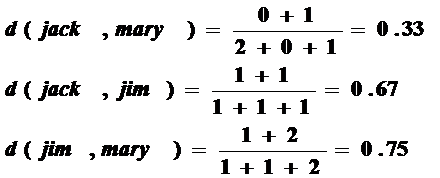### Similarity and Dissimilarity

• Similarity
• Numerical measure of how alike two data objects are
• Value is higher when objects are more alike
• Often falls in the range [0,1]
• Dissimilarity (e.g., distance)
• Numerical measure of how different two data objects are
• Lower when objects are more alike
• Minimum dissimilarity is often 0
• Upper limit varies
• Proximity refers to a similarity or dissimilarity

### Data Matrix and Dissimilarity Matrix

 Data matrix n data points with p dimensions Two modes Dissimilarity matrix n data points, but registers only the distance A triangular matrix Single mode

### Proximity Measure for Nominal Attributes

• Can take 2 or more states, e.g., red, yellow, blue, green (generalization of a binary attribute)
• Method 1: Simple matching
• m : # of matches, p : total # of variables

$d(i,j)=\frac{p-m}{p}$

• Method 2: Use a large number of binary attributes
• creating a new binary attribute for each of the M nominal states

### Proximity Measure for Binary Attributes

• A contingency table for binary data

• Distance measure for symmetric binary variables:
$d(i,j)=\frac{r+s}{q+r+s+t}$
• Distance measure for asymmetric binary variables:

$d(i,j)=\frac{r+s}{q+r+s}$

• Jaccard coefficient (similarity measure for asymmetric binary variables):

$sim_{Jaccard}(i,j)=\frac{q}{q+r+s}$

• Note: Jaccard coefficient is the same as “coherence”:

$coherence(i,j)=\frac{sup(i,j)}{sup(i)+sup(j)-sup(i,j)}=\frac{q}{(q+r)(q+s)-q}$

### Dissimilarity between Binary Variables

• Example

• Gender is a symmetric attribute
• The remaining attributes are asymmetric binary
• Let the values Y and P be 1, and the value N 0### Standardizing Numeric Data

• Z-score:

$z=\frac{x-\mu}{\sigma }$

• X: raw score to be standardized, μ: mean of the population, σ: standard deviation
• the distance between the raw score and the population mean in units of the standard deviation
• negative when the raw score is below the mean, “+” when above
• An alternative way: Calculate the mean absolute deviation, where

$m_{f}= \frac{1}{n}(x_{1f}+x_{2f}+...+x_{nf})$

• standardized measure (z-score):

$z_{if}=\frac{(x_{if}-m_{f})}{S_{f}}$

• Using mean absolute deviation is more robust than using standard deviation
$s_{f}=\frac{1}{n} (|x_{1f}-m_{f}|+|x_{2f}-m_{f}|+...+|x_{nf}-m_{f}|)$

### Distance on Numeric Data: Minkowski Distance

• Minkowski distance: A popular distance measure

where i = (xi1, xi2, …, xip) and j = (xj1, xj2, …, xjp) are two p-dimensional data objects, and h is the order (the distance so defined is also called L-h norm)

• Properties
• d(i, j) > 0 if i ≠ j, and d(i, i) = 0 (Positive definiteness)
• d(i, j) = d(j, i) (Symmetry)
• d(i, j) ≤ d(i, k) + d(k, j) (Triangle Inequality)
• A distance that satisfies these properties is a metric

### Special Cases of Minkowski Distance

• h = 1: Manhattan (city block, L1 norm) distance
• E.g., the Hamming distance: the number of bits that are different between two binary vectors
$d(i,j)=|x_{i1}-x_{j1}|+|x_{i2}-x_{j2}|+...+|x_{ip}-x_{jp}|$

• h = 2: (L2 norm) Euclidean distance

$d(i,j)=\sqrt{(|x_{i1}-x_{j1}|^2+|x_{i2}-x_{j2}|^2+...+|x_{ip}-x_{jp}|^2)}$

• h →≈ . “supremum” (Lmax norm, L norm) distance.
• This is the maximum difference between any component (attribute) of the vectors
$d(i,j)=\lim_{h\rightarrow \infty }(\sum_{f=1}^{p}|x_{if}-x_{jf}|^{h})^\frac{1}{h} =max_{f}^{p}|x_{if}-x_{jf}|$

### Ordinal Variables

• An ordinal variable can be discrete or continuous
• Order is important, e.g., rank
• Can be treated like interval-scaled
• replace xif by their rank

$r_{if} \epsilon \left \{ 1,...,M_{f} \right \}$

• map the range of each variable onto [0, 1] by replacing i-th object in the f-th variable by
• compute the dissimilarity using methods for interval-scaled variables
$z_{if} = \frac{r_{if}-1}{M_{f}-1}$

### Attributes of Mixed Type

• A database may contain all attribute types
• Nominal, symmetric binary, asymmetric binary, numeric, ordinal
• One may use a weighted formula to combine their effects

$d(i,j) = \frac{\sum_{f=1}^{p} \delta _{ij}^{(f)} d_{ij}^{(f)}}{\sum_{f=1}^{p} \delta _{ij}^{(f)}}$

• f is binary or nominal:
• dij(f) = 0 if xif = xjf , or dij(f) = 1 otherwise
• f is numeric: use the normalized distance
• f is ordinal
• Compute ranks rif and
• Treat zif as interval-scaled
$z_{if} = \frac{r_{if}-1}{M_{f}-1}$

### Cosine Similarity

• A document can be represented by thousands of attributes, each recording the frequency of a particular word (such as keywords) or phrase in the document.

• Other vector objects: gene features in micro-arrays, …
• Applications: information retrieval, biologic taxonomy, gene feature mapping, ...
• Cosine measure: If d1 and d2 are two vectors (e.g., term-frequency vectors), then
cos(d1, d2) =  (d1 ⋅ d2) /||d1|| ||d2|| ,
where ⋅ indicates vector dot product, ||d||: the length of vector d

### Example: Cosine Similarity

• cos(d1, d2) = (d1d2) / ||d1|| ||d2|| ,
where ⋅ indicates vector dot product, ||d|: the length of vector d
• Ex: Find the similarity between documents 1 and 2.
d1 = (5, 0, 3, 0, 2, 0, 0, 2, 0, 0)
d2 = (3, 0, 2, 0, 1, 1, 0, 1, 0, 1)
d1 d2 = 5*3+0*0+3*2+0*0+2*1+0*1+0*1+2*1+0*0+0*1 = 25
||d1|| = (5*5+0*0+3*3+0*0+2*2+0*0+0*0+2*2+0*0+0*0)0.5 = (42) 0.5 = 6.481
||d2|| = (3*3+0*0+2*2+0*0+1*1+1*1+0*0+1*1+0*0+1*1)0.5 = (17) 0.5 = 4.12
cos(d1, d2 ) = 0.94